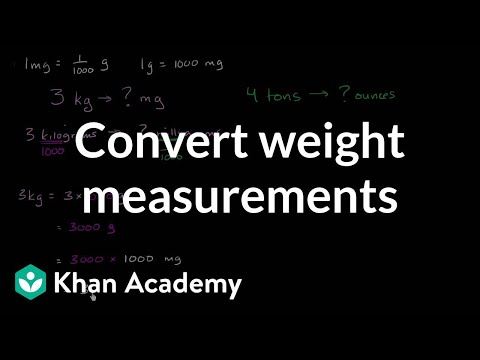COVID-19 presents an unprecedented challenge for teachers, students, and parents. Explore resources from IBM and partners here.
Video

# Multi-step unit conversion examples (metric) (Full video)

Khan Academy

## Supporting grades: 4

Description: Sal solves multi-step unit conversion examples with metric units of measure. So what I wanna do is, I'm gonna convert both of these to grams, and then add 'em up to get the combined weight. So this is going to be 1,000 times 1.38 is 1,380 grams, which I could've just denoted with a G, but now let's add those together. This is the first package, and then the second package is 720 grams, and so if I were to add, this is going to be, let's see, got a zero, 10, well let's see, four plus seven is 11, and then one plus one is two.

You must log inorsign upif you want to:*

*Teacher Advisor is 100% free.

### Other videos you might be interested in### Converting metric units of length (Full video)

#### Khan Academy